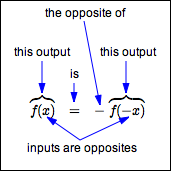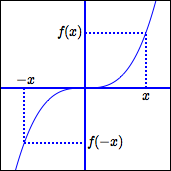﻿ Even and Odd Functions

# EVEN AND ODD FUNCTIONS

• PRACTICE (online exercises and printable worksheets)

## EVEN FUNCTIONS

DEFINITION even functions
 A function $\,f\,$ is even if and only if for all $\,x\,$ in the domain of $\,f\,$, $\,f(x) = f(-x)\,$.
for even functions:
when inputs are opposites, the corresponding outputs are the same• Recall that $\,x\,$ and $\,-x\,$ are opposites.
When $\,x\,$ is the input, $\,f(x)\,$ is the output.
When $\,-x\,$ is the input, $\,f(-x)\,$ is the output.
For even functions, these two outputs must be equal.
• Note: A function $\,f\,$ is even if and only if the graph of $\,f\,$ is symmetric about the $\,y\,$-axis.
• Note:   $\,y = x^2\,$,   $\,y = x^4\,$,   $\,y = x^6\,$,   $\,y = x^8\,$, etc., are all even functions.
Thus, power functions with even powers are even functions!
So, the name ‘even’ seems reasonable.
• There are lots of other even functions.
For example, $\,y = |x|\,$ and $\,y = \cos(x)\,$ are even functions.
• Is   $\,g(t) = t^2 - t^4\,$   an even function?
You must investigate $\,g(-t)\,$, and compare it with $\,g(t)\,$, as follows:

 $g(-t)$ $=$ $(-t)^2 - (-t)^4$ definition of $\,g\,$; function evaluation $=$ $t^2 - t^4$ simplify $=$ $g(t)$ definition of $\,g$

Thus, $\,g\,$ is an even function.

## ODD FUNCTIONS

DEFINITION odd functions
 A function $\,f\,$ is odd if and only if for all $\,x\,$ in the domain of $\,f\,$, $\,f(x) = -f(-x)\,$.
for odd functions:
when inputs are opposites, the corresponding outputs are opposites• Recall that $\,x\,$ and $\,-x\,$ are opposites.
When $\,x\,$ is the input, $\,f(x)\,$ is the output.
When $\,-x\,$ is the input, $\,f(-x)\,$ is the output.
For odd functions, these two outputs must be opposites.
• Observe that ‘$\,f(x) = -f(-x)\,$’ is equivalent to ‘$\,f(-x) = -f(x)\,$’ (multiply both sides by $\,-1\,$ and switch sides).
Sometimes, the definition is given using this equivalent characterization.
• Note: A function $\,f\,$ is odd if and only if the graph of $\,f\,$ is symmetric about the origin.
• Note:   $\,y = x\,$,   $\,y = x^3\,$,   $\,y = x^5\,$,   $\,y = x^7\,$,   $\,y = x^9\,$, etc., are all odd functions.
Thus, power functions with odd powers are odd functions!
So, the name ‘odd’ seems reasonable.
• There are lots of other odd functions.
For example, $\displaystyle \,y = \frac{1}{x}\,$ and $\,y = \sin(x)\,$ are odd functions.
• Is   $\,g(x) = x^3 - 1\,$   an odd function?
That is, are $\,g(-x)\,$ and $\,g(x)\,$ opposites?
That is, are $\,g(-x)\,$ and $\,-g(x)\,$ equal?

 $g(-x) = (-x)^3 - 1 = -x^3 - 1$ find $\,g(-x)\,$:   definition of $\,g\,$;   function evaluation $-g(x) = -(x^3-1) = -x^3 + 1$ find the opposite of $\,g(x)\,$:   definition of $\,g\,$;   the distributive law

Since $\,g(-x)\,$ and $\,-g(x)\,$ are different, $\,g\,$ is not an odd function.
• If an odd function is defined at zero, then its graph must pass through the origin.
Why?
If $\,f\,$ is odd and defined at zero, then:

 $f(x) = -f(-x)$ requirement for an odd function $f(0) = -f(-0)$ let $\,x = 0$ $f(0) = -f(0)$ the opposite of zero is zero $2f(0) = 0$ add $\,f(0)\,$ to both sides $f(0) = 0$ divide both sides by $\,2$
Master the ideas from this section